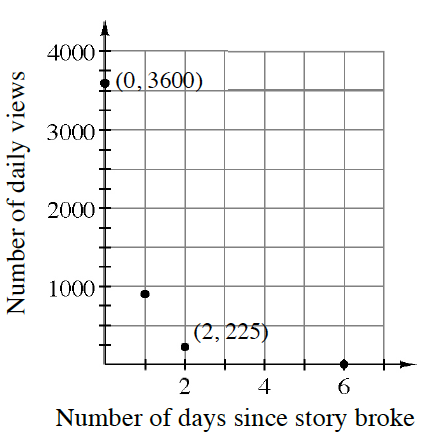### Home > PC3 > Chapter Ch8 > Lesson 8.3.1 > Problem8-93

8-93.

When a news story breaks, it can generate a lot of interest, but it does not take long before another story diverts that interest. At right is a graph depicting the number of daily views for a news story about Syria and the United Nations.

1. Write an equation to model the situation.

This is exponential decay. Write an equation of the form $y = a·b^{x}$.

2. If this model is typical of how interest declines for a news story, what is the half-life of interest in the story?

Since there are $3600$ initial views, determine how long until there are $1800$ views.

3. Write an equation that models this phenomenon for any initial value of views ($I$).

See the hint in part (a). Then let $a = I$.